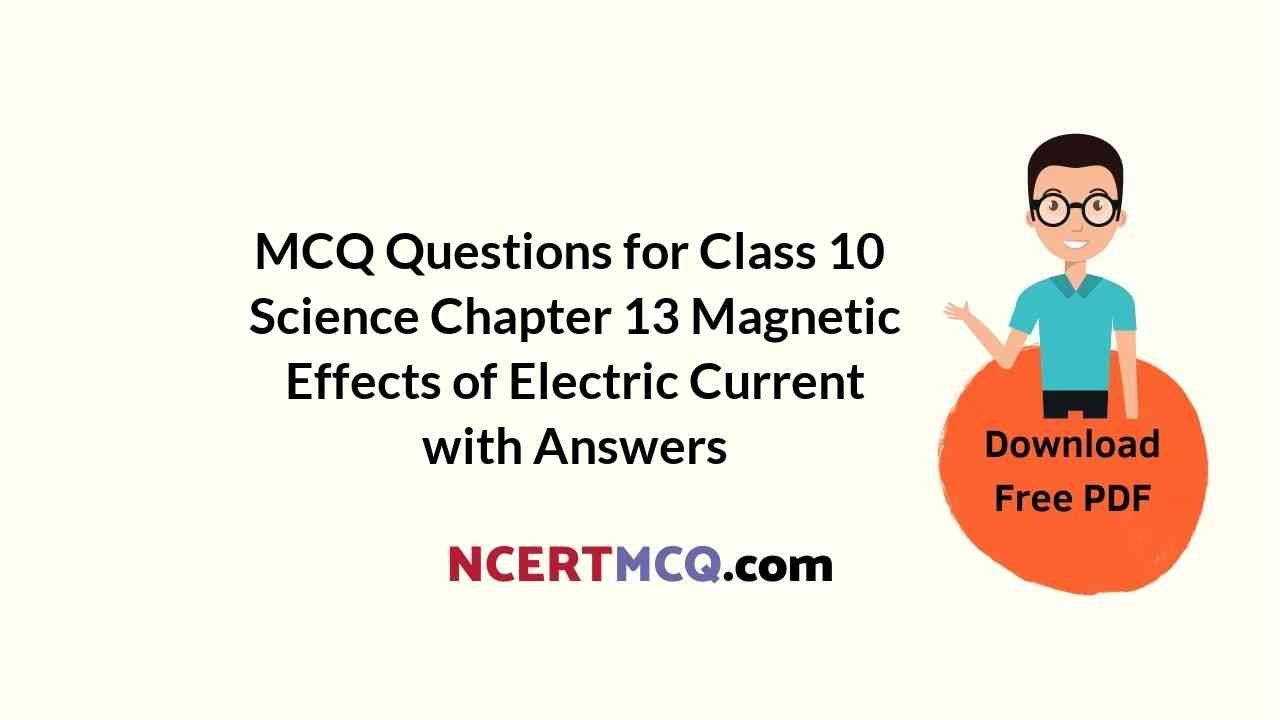Students can also read MCQ Questions for Class 10 Science Chapter 13 Magnetic Effects of Electric Current Questions with Answers hope will definitely help for your board exams. https://ncertmcq.com/mcq-questions-for-class-10-science-with-answers/

## Magnetic Effects of Electric Current Class 10 MCQs Questions with Answers

Magnetic Effect Of Electric Current Class 10 MCQ Question 1.
If the key in the arrangement as shown below is taken out (the circuit is made open) and magnetic field lines are drawn over the horizontal plane ABCD, the lines are: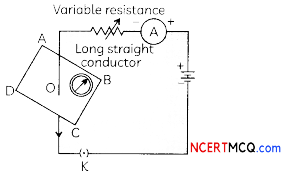(a) Concentric circles
(b) Elliptical in shape
(c) Straight lines parallel to each other
(d) Concentric circles near the point O but of elliptical shapes as we go away from it
(c) Straight lines parallel to each other

Explanation: If the circuit connection breaks by taking the key out, then no current will flow through the wire and no magnetic field will exist due to the conductor.

Therefore, at the point O, there will be only Earth’s magnetic field and they are straight lines parallel to each other.

Magnetic Effect Of Electric Current MCQ Question 2.
A current-carrying conductor is held in exactly vertical direction. In order to produce a clockwise magnetic field around the conductor, the current should passed in the conductor:
(a) from top towards bottom
(b) from left towards right
(c) from bottom towards top
(d) from right towards left

Class 10 Magnetic Effect Of Electric Current MCQ Question 3.
A circular loop placed in a plane perpendicular to the plane of paper carries a current when the key is on. The current as seen from points A and B (in the plane of paper and on the axis of the coil) is anticlockwise and clockwise respectively. The magnetic field lines point from B to A. The N-pole of the resultant magnet is on the face close to: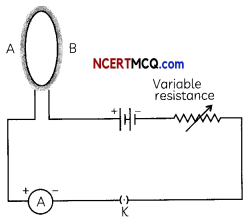(a) A
(b) B
(c) A if the current is small and B if the current is large
(d) B if the current is small and A if the current is largeMCQ On Magnetic Effect Of Electric Current Question 4.
A uniform magnetic field exists in the plane of paper pointing from left to right as shown in the figure. In the field, an electron and a proton move as shown. The electron and the proton experience: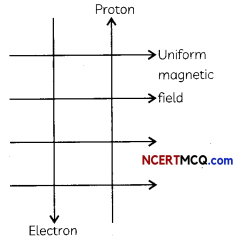(a) forces both pointing into the plane of paper
(b) forces both pointing out of the plane of paper
(c) forces pointing into the plane of paper and out of the plane of paper, respectively
(d) forces pointing opposite and along the direction of the uniform magnetic field respectively
(a) forces both pointing into the plane of paper

Explanation: Apply Fleming’s left hand rule and considering that the flow of current in the direction of the movement of proton and in the direction opposite to the flow of electrons. So, the current due to both electron and proton will be in the same direction because of which, the forces acting on both will be in the same direction. By Fleming’s Left hand rule, the direction of force is pointing into the plane of paper.

Magnetic Effects Of Electric Current Class 10 MCQ Question 5.
For a current in a long straight solenoid N and S-poles are created at the two ends. Among the following statements, the incorrect statement is:
(a) The field lines inside the solenoid are in the form of straight lines, which indicates that the magnetic field is the same at all points inside the solenoid
(b) The strong magnetic field produced inside the solenoid can be used to magnetize a piece of magnetic material Like soft iron, when placed inside the coil
(c) The pattern of the magnetic field associated with the solenoid is different from the pattern of the magnetic field around a bar magnet
(d) The N and S-poles exchange position when the direction of current through the solenoid is reversed.

Magnetic Effects Of Electric Current MCQ Question 6.
An electron moves with a speed v along positive direction of the x-axis. If a magnetic field B acts along the positive y-direction, then the force on the electron will act along:
(a) x-axis
(b) y-axis
(c) -ve z-direction
(d) +ve z-direction [Diksha]
(c) -ve z-direction

Explanation: As electron is moving in positive x-direction, then, according to Maxwell’s right-hand thumb rule, the current is moving in negative x-direction and the magnetic field acts on positive y-direction.

Thus, the thumb will be in negative z-direction, which is the direction of force.

MCQ Of Magnetic Effect Of Electric Current Question 7.
Which of the following is not attracted by a magnet:
(a) Steel
(b) Cobalt
(c) Brass
(d) NickelCh 13 Science Class 10 MCQ Question 8.
The magnetic field lines:
(a) intersect at right angles to one another
(b) interest at an angle 45° to each another
(c) do not cross one another
(d) cross at an angle of 60° to one another
(c) do not cross one another

Explanation: The magnetic field lines do not cross one another because the resultant force at any point on the north pole can only be in one direction. This is impossible if the Lines intersect

Related Theory
If two magnetic field lines are found to cross each their, it means that at the point of intersection, the compass needle would point towards two directions. which is not possible.

Magnetism Class 10 MCQ Question 9.
The magnetic field inside a long straight solenoid carrying current is
(a) uneven
(b) zero
(c) same at aLL points
(d) different at aU Points
(c) same at all points

Explanation: The magnetic field lines inside a long straight solenoid carrying current is in the form of parallel straight tines which indicates that the magnetic field is some at oIL points inside the solenoid.

MCQs On Magnetic Effects Of Electric Current Class 10 Question 10.
To convert an AC generator into DC generator:
(a) Split ring type commutator must be used
(b) SLIp rings and brushes must be used
(c) A stronger magnetic fieLd has to be used
(d) A rectangular wire Loop has to be used

Magnetic Effect Of Electric Current MCQs Question 11.
The most important safety method used for protecting home appliances from short-circuiting or overloading is:
(a) Earthing
(b) Use of fuse
(c) Use of stabiLizers
(d) Use of electric meterClass 10 Science Ch 13 MCQ Question 12.
Which of the given statements are incorrect regarding magnetic field Unes?
(I) The direction of magnetic field at a point is taken to be the direction In which the south pole of a magnetic compass needle points.
(II) Magnetic field Lines are closed curves.
(III) If magnetic field Lines are parallel and equidistant, they represent zero-field strength.
(IV)Relative strength of magnetic field is shown by the degree of closeness of the field lines.
(a) Both (I) and (II)
(b) Both (II) and (III)
(c) Both (I) and (III)
(d) Both (II) and (IV)
(c) Both (I) and (III)

Explanation: The direction of magnetic field at a point is taken to be the direction in which the north pole of a magnetic compass needle points. If magnetic field lines are parallel and equidistant, they represent uniform field strength.

MCQ Questions On Magnetic Effects Of Electric Current Question 13.
The north pole of a long bar magnet was pushed slowly into a short solenoid connected to a galvanometer. The magnet was held stationary for a few seconds with the north pole in the middle of the solenoid and then withdrawn rapidly.
Select the correct observations:
(I) The maximum deflection of the galvanometer will be observed when the magnet was moving out of the solenoid.
(II) The maximum deflection of the galvanometer will be observed when the magnet was moving slowly into of the solenoid.
(III) The minimum deflection of the galvanometer will be observed when the magnet was at rest inside the solenoid.
(IV) The minimum deflection of the galvanometer will be observed when the magnet was moving towards the solenoid.
(a) Both (I) and (III)
(b) Both (II) and (III)
(c) Both (I) and (IV)
(d) Both (II) and (IV)
(a) Both (I) and (III)

Explanation: The maximum deflection of the galvanometer will be observed when the magnet was moving out of the solenoid as maximum current is induced in the coil due to the relative motion between the solenoid coil and the magnet. Whereas, the minimum deflection of the galvanometer will be observed when the magnet was at rest inside the solenoid as no current is induced in the coil.

MCQs On Magnetic Effect Of Electric Current Question 14.
A student places some iron fillings around a magnet. The iron fillings arrange themselves as shown in image below.Four points labelled A, B, C and D have been marked in the figure.
Select the correct statement regarding the strength of magnetic field at these points:
(I) The magnetic field will be strongest at A.
(II) The magnetic field will be strongest at B.
(III) The magnetic field will be weakest at C.
(IV)The magnetic field will be weakest at D.
(a) Both (I) and (III)
(b) Both (II) and (III)
(c) Both (I) and (IV)
(d) Both (II) and (IV)MCQ Of Magnetic Effect Of Electric Current Class 10 Question 15.
The figure below shows an electric generator.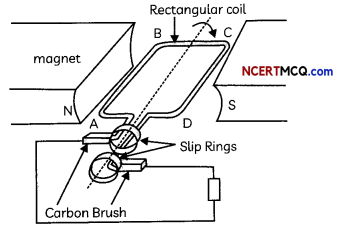The coil ABCD is rotated and the direction of induced current is noted initially and when coil completes half cycle of the rotation.
Select the row containing correct direction of induced current in the coil ABCD.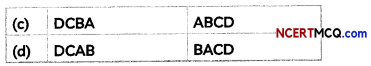(a) Initially when coil ABCD is rotated ABCD; when coil complete is half cycle of rotation DCBA

Explanation: Length AB of the coil ABCD is moving upwards and the magnetic field acts from left to right. Hence, according to Fleming’s right-hand rule, the direction of induced current will be from A to B. And the direction of induced current in the length CD will be from C to D. The direction of current in the coil is ABCD. After half a rotation, length AB starts moving down whereas length CD starts moving upward. The direction of the induced current in the coil gets reversed as DCBA.

Question 16.
Study the graphs (A) and (B) shown below and select the row containing incorrect information from the table.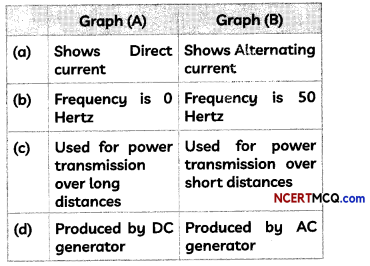Question 17.
The magnetic field lines inside a current-carrying solenoid are in the form of parallel straight lines which indicate that:
(a) The magnetic field is zero inside the solenoid.
(b) The field is uniform inside the solenoid.
(d) The field is non-uniform inside the solenoid.
(d) The field is very strong inside the solenoid.
(b) The field is uniform inside the solenoid.

Explanation: The magnetic field lines inside a current-carrying solenoid are in the form of parallel straight lines which indicate that the field is uniform inside the solenoid.

Question 18.
A current-carrying conductor is held in the vertical direction. In order to produce a clockwise magnetic field around the conductor, the current should be passed in the conductor:
(a) From top to bottom
(b) From left to right
(c) From bottom to top
(d) From right to left
(a) From top to bottom

Explanation: By applying right-hand thumb rule, we note that in order to produce a clockwise magnetic field around the conductor, the current should be passed from top to bottom in the conductor as shown: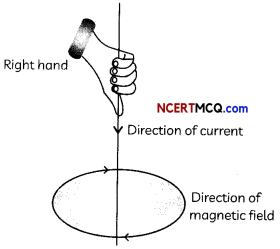Question 19.
An induced emf is produced when a magnet is moved into a coil. The magnitude of induced emf does not depend on:
(a) The number of turns of the coil
(b) The speed with which the magnet is moved
(c) The strength of the magnet
(d) The resistivity of the wire of the coil

Question 20.
Imagine that a negative charge is moving towards a person. The direction of magnetic field lines will be in:
(a) Clockwise direction
(b) Anticlockwise direction
(c) Vertically upward direction
(d) Vertically downward direction

### Assertion Reasoning questions Class 10 Science Chapter 13

For the following questions, two statements are given one labeled Assertion (A) and the other labeled Reason (R). Select the correct Answer to these questions from the codes (a), (b), (c), and (d) as given below.
(a) Both (A) and (R) are true, and (R) is correct explanation of the assertion.
(b) Both (A) and (R) are true, but (R) is not the correct explanation of the assertion.
(c) (A) is true, but (R) is false.
(d) (A) is flase, but R is true.

Question 21.
Assertion (A): The energy of charged particle moving in a uniform magnetic field does not change.
Reason (R): Work done by magnetic field on the charge is zero.
(a) Both (A) and (R) are true and (R) is the correct explanation of assertion.

Question 22.
Assertion (A): The compass placed near the current-carrying wire remains stationary.
Reason (R): The current flowing through a wire gives rise to a magnetic field.
(d) (A) is wrong but (R) is correct.

Explanation: The compass when placed near the current-carrying wire gets deflected because the current flowing through the wire gives rise to a magnetic field.Question 23.
Assertion (A): The direction of force acting on a current carrying conductor placed in a magnetic field gets reversed on reversing the direction of current flowing through the conductor.
Reason (R): Magnitude of force is highest when direction of current is parallel to direction of magnetic field.

Question 24.
Assertion (A): Current can be induced in a coil by changing the magnetic field around it.
Reason (R): A galvanometer connected to a coil can deflect either to the left or right of the zero marks.
(b) Both (A) and (R) are true but (R) is not the correct explanation of assertion. Explanation: Voltage and hence current is induced in a coil due to a changing magnetic field and this process is known as electromagnetic induction.

A galvanometer is a device that detects the presence of current by deflecting the needle to one side of the zero mark, but this does not explain the current induced in the coil.

Competency-Based Questions (CBQs)

Question 1.
The space surrounding a magnet in which magnetic force is exerted, is called a magnetic field. The direction of magnetic field lines at a place can be determined by using a compass needle. A compass needle placed near a magnet gets deflected due to the magnetic force exerted by the magnet.

The north end of the needle of the compass indicates the direction of magnetic field at the point where it is placed. When the magnet shown in the diagram below is moving towards the coil, the galvanometer gives a reading to the right.(A) What is the name of the effect being produced by the moving magnet?
(B) State what happens to the needle shown on the galvanometer when the magnet is moving away from the coil.
(C) If the magnet is moved towards the coil at a great speed, state two changes that you would notice in the galvanometer.
(D) List three sources of magnetic fields.

Question 2.
Happy’s friend once got a bicycle fitted with a dynamo to school. Happy was quite thrilled to see a cycle fitted with a dynamo and decided to find out more about this. A bottle dynamo or sidewall dynamo is a small electrical generator for bicycles employed to power a bicycle’s lights. When the bicycle is in motion and the dynamo roller is engaged, electricity is generated as the tire spins the roller.Two other dynamo systems used on bicycles are hub dynamos and bottom bracket dynamos.Fleming’s Right-hand rule gives:
(a) magnitude of the induced current.
(b) magnitude of the magnetic field
(c) direction of the induced current
(d) both, direction and magnitude of the induced currentQuestion 3.
Vaibhav performed an activity to observe an important breakthrough made by the English Physicist faraday. He took a coil of wire AB having a large number of turns and connected the ends of the coil to a galvanometer. He observed a momentary deflection in the needle of the galvanometer, say to the right, when the North pole of a strong magnet was moved towards the end B of the coil.
(A) Name the phenomenon discovered by Faraday.
The phenomenon was Electromagnetic induction.

(B) Why does Vaibhav observe momentary deflection in the galvanometer?
This is due to induced current in the coil as a result of changing magnetic field associated with the coil.

(C) What happens to the deflection in the galvanometer when the motion of the magnet stops?

(D) What will be observed when the north pole of the magnet is withdrawn away from the coil?
We observe that the galvanometer is deflected toward the left, showing that the current is now set up in the direction opposite to the first

Question 4.
Transmission lines are sets of wires that carry electric power from generating plants to the substations that deliver power to customers. At a generating plant, electric power is “stepped up” to several thousand volts by a transformer and delivered to the transmission line. When Kumar visited his grandmother’s home, he was quite surprised to see the transmission lines going right above their colony!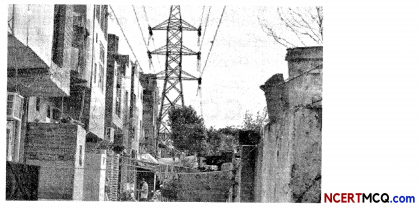A constant current flows in a horizontal wire in the plane of the paper from east to west as shown in the figure. The direction of a magnetic field at a point will be north to south: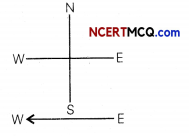(a) directly above the wire
(b) directly below the wire
(c) at a point located in the plane of the paper on the north side of the wire
(d) at a point located in the plane of the paper on the south side of the wire
(b) directly below the wire

Explanation: If we observe the direction of the magnetic field by applying the right hand thumb rule, then we will find that the directior of the magnetic field is from north to south below the wire

Question 5.
Fix a sheet of white paper on a drawing board using some adhesive material. Place a bar magnet in the centre of it. Sprinkle some iron filings uniformly around the bar magnet as shown in figure below. Now tap the board gently.In the next activity, take a small compass and a bar magnet. Place the magnet on a sheet of white paper fixed on a drawing board, using some adhesive material. Mark the boundary of the magnet. Place the compass near the north pole of the magnet. Mark the position of two ends of the needle. Now move the needle to a new position such that its south pole occupies the position previously occupied by its north pole. In this way, proceed step by step till you reach the south pole of the magnet as shown in Fig. below.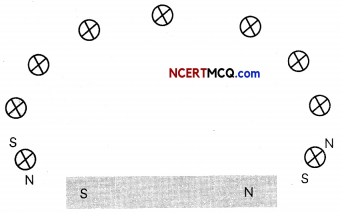Join the points marked on the paper by a smooth curve. Repeat the above procedure and draw as many lines as you can. Observe the deflection in the compass needle as you move it along a field line.
(A) Select the correct observations and conclusions when cardboard is gently tapped in the first activity:
(I) The iron filings arrange themselves in a pattern.
(II) The magnet experiences a force as the iron atoms exert its influence on it.
(III) The force experienced by the magnet makes iron filings to arrange in a pattern.
(IV) The region surrounding a magnet, in which the force of the magnet can be detected, is said to have a magnetic field.
(a) Both (I) and (II)
(b) Both (III) and (III)
(c) Both (I) and (IV)
(d) Both (II) and (IV)

(B) The lines along which the iron filings align themselves represent:
(a) Magnetic field lines.
(b) Electric field lines
(c) Magnetic potential
(d) Electric potential
(a) Magnetic field lines

Explanation: The magnetic field lines are the imaginary lines drawn around a magnet that represent the m etic field around a magnet.

(C) Select the row containing the correct observation when a small compass is placed near the bar magnet in the second activity:

 North Pole of Compass Needle South Pole of Compass Needle (a) It points towards the North pole of the magnet It points towards the South pole of the magnet (b) It points towards the North pole of the magnet It points away from the North pole of the magnet (c) It points away from the South pole of the magnet It points away from the North pole of the magnet (d) It points away from the North pole of the magnet It points towards the North pole of the magnet

(d) North Pole of compass needle: It points away from the North Pole of the magnet: South Pole Compass needle: it Points towards the North Pole of the Magnet.

Explanation: The small compass is actuaLly a magnetic compass needle. Its North pole points away from the North pole of the bar magnet and its South pole points towards the North pole of the bar magnet as like poles repel each other and unlike poles attract each other.

(D) When the compass needle is moved along a field line, the deflection in the compass:
(a) First increases and then decreases.
(b) Increases as the needle is moved towards the poles.
(c) Decreases as the needle is moved towards the poles.
(d) Remains constant
(E) Given below are four figures showing the direction of magnetic field lines around a bar magnet. Select the option showing the correct direction:Question 6.
Vikram had gone to his cousin’s apartments to celebrate holi. While climbing the stairs he observed that all the electric meters and main switches were installed on one side of the stair case. On each meter box, apart from other information, the following was also written: kWh meter, 220VAC.How many times does AC used in India change direction in one second?
The frequency of alternating current in India is 50 Hertz. This means that the AC changes current every 1/100 second.

Question 7.
Kritika once observed a caling bell on the wall just outside the principal’s room. Although she knew its purpose, she wanted to know the construction and working of an electric calling bell, which is a mechanical or electronic bell that functions by means of an electromagnet.(a) Will decrease
(b) Will increase
(c) Will become zero
(d) Will remain the sameQuestion 8.
Take a coil of wire AB having a large number of turns. Connect the ends of the coil to a galvanometer as shown in Fig. (a) below.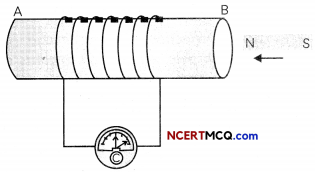(a)
Take a strong bar magnet and move its north pole towards the end B of the coil. Observe the deflection in the galvanometer towards right. Now withdraw the north pole of the magnet away from the coil. Next, place the magnet stationary at a point near to the coil, keeping its north pole towards the end B of the coil. First move the coil towards the north pole of the magnet and then away from the magnet. Observe the deflection of the galvanometer in both cases.

Next, take two different coils of copper wire having large number of turns (say 50 and 100 turns respectively). Insert them over a non-conducting cylindrical roll, as shown in Fig. below. Connect the coil-1, having larger number of turns, in series with a battery and a plug key. Also connect the other coil-2 with a galvanometer as shown in fig. (b) below.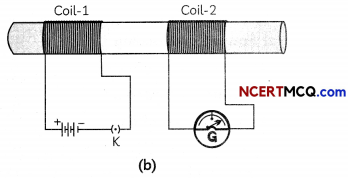Plug in the key. Observe the deflection in galvanometer. Next, disconnect coil-1 from the battery. Note your observations.
(A) Select the correct observations when a strong bar magnet is taken and its north pole is moved near the coil in the first activity:
(I) There is a momentary deflection in the needle of the galvanometer, say to the right, when the north pole of a strong bar magnet is moved towards the end B of the coil.
(II) Momentary deflection in the galvanometer needle indicates the presence of a force in the coil AB.
(III) The deflection in the galvanometer increases the moment the motion of the magnet stops.
(IV) When the north pole of the magnet is withdrawn away from the coil, the galvanometer is deflected toward the left.
(a) Both (I) and (III)
(b) Both (I) and (IV)
(c) Both (II) and (III)
(d) Both (III) and (IV)
(b) Both (I) and (IV)

Explanation: Momentary deflection in the galvanometer needle indicates the presence of a current in the coil AB. The deflection in the galvanometer becomes zero the moment the motionof the magnet stops as no current is induced in the coil now.

(B) Refer to fig. (a) above. Place the magnet stationary at a point near to the coil, keeping its north pole towards the end
B of the coil.
Select the row containing incorrect observation:

 Movement of coil Deflection of the galvanometer needle (a) Coil is moved towards the North pole of the magnet Towards right (b) Coil is moved away the North pole of the magnet Towards left (c) Both coil and magnet are stationary Momentary deflection (d) Both coil and magnet are stationary No deflection

(c) Movement of coil: Both coil and magnet are stationary; Deflection of galvanometer needle: Momentary deflection

Explanation: When the coil is moved towards the north pole of the galvanometer, the galvanometer needle deflects towards right and when the coil is moved away from the north pole of the magnet, the galvanometer needle deflects in the opposite direction towards right. This is because the induced current is now set up in the direction opposite to the first.

However, when both the coil and magnet are stationary, there is no induced current in the coil and hence no deflection in the needle of the galvanometer.

(C) In the first activity, what would be observed if the south pole of the magnet was moved away from the end B of the
coil?
(a) Deflection in same direction as when north pole was moved towards end B of the coil, i.e., towards right.
(b) Deflection in opposite direction as when north pole was moved towards end B of the coil, i.e., towards left.
(c) First deflection towards right and then towards left.
(d) No deflection in galvanometer needle.
(a) Deflection in same direction as when north pole was moved towards end B of the coil, i.e., towards right.

Explanation: When the south pole of magnet is moved away from the end B of the coil, the current will be induced in the coil in the same direction as north pole moving towards the end B of the magnet. Therefore, deflection of galvanometer needle will be in the same direction which is towards right.

(D) Select the incorrect observation when the key is plugged “in” in the second activity:
(a) The needle of the galvanometer instantly jumps to one side and quickly returns to zero, indicating a momentary current in coil-2.
(b) When the battery is disconnected from the coil, there is a momentary deflection of the galvanometer needle in opposite side.
(c) The needle of the galvanometer jumps to one side till the key is plugged “in” indicating that current is induced in coil-2.
(d) As soon as the current in coil-1 reaches either a steady value or zero, the galvanometer in coil-2 shows no deflection.

(E) Cause of induced current in coil 2 in fig (b) is:
(a) Change in magnetic field lines associated with the secondary coil.
(b) Change in magnetic field lines associated with the primary coil.
(c) Change in magnetic force associated with the secondary coil.
(d) Change in magnetic force associated with the primary coil.Question 9.
Paras was keenly observing the electrician connecting the new electric cooler at their home. He observed that apart from the two wires, there was a third wire which he connected at the plug, which was a three pin plug. He came to know the third wire is the earth wire.The earth wire is used as a safety measure to:
(b) Prevent shortcircuiting
(c) Prevent electric shock
(d) Voltage fluctuations

Question 10.
When the mason working at Smriti’s house, turned on his tile cutter connected to the switchboard of the living room, there was a fire in the plug. Immediately, her father switched off the electric mains supply and the fire was prevented from spreading further.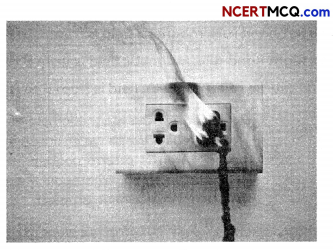State the possible reason for such a situation leading to an electric fire.
One possible reason for this kind of electric fire is that the power rating of the tile cutter was higher than the current carrying limit of the electric circuit to which it was connected. This led to the damaging of the insulation of the connecting wires and hence short circuiting took place.Question 11.
Valves are mechanical devices designed to control the flow of liquid and gases. Many valves are manually operated. Electrically operated valves are known as solenoid valves. A solenoid valve consists of two basic units: an assembly of the solenoid (the electromagnet) and plunger (the core), and a valve containing an opening (orifice) in which a disc or plug is positioned to control the flow of fluid. The valve is opened or closed by the movement of the magnetic plunger. When the coil is energized, the plunger is drawn into the solenoid (electromagnet) and flow through the orifice is allowed. The valve returns automatically to its original position when current ceases due to the pressure of spring and flow through the orifice is restricted.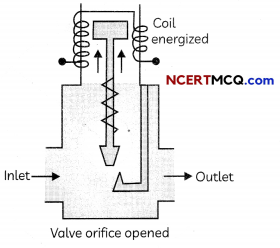(A) Which of the following statements is correct regarding solenoid valve?
(a) Solenoid valves are mechanically operated valves.
(b) A solenoid valve is opened or closed by the movement of the magnetic plunger.
(c) Flow through the orifice is allowed when no current flows through the solenoid coil.
(d) Flow through the orifice is restricted when current flows through the solenoid coil.

(B) Select the incorrect statements regarding the magnetic field in a current carrying solenoid as shown above:
(I) The pattern of the magnetic field lines around a current-carrying sole¬noid is similar to the magnetic field around a bar magnet.
(II) One end of the solenoid behaves as a positive charge and the other end behaves as a negative charge.
(III) The field lines inside the solenoid are in the form of curved lines.
(IV) The magnetic field is uniform inside the solenoid.
(a) Both (I) and (II)
(b) Both (II) and (III)
(c) Both (III) and (IV)
(d) Both (I) and (IV)
(b) Both (II) and (III)

Explanation: One end of the solenoid be-haves as a magnetic north pole and the other end behaves as a magnetic south pole. The field lines inside the solenoid are in the form of parallel straight lines.

(C) The figure below shows the lengthwise section of a current carrying solenoid, where indicates that current is entering into the page and indicates that current is emerging out of the page:The table below gives the identification of the ends A and B of the solenoid as North or South pole and the justification for the answer. Select the row containing the correct identification of the ends A and B and the correct justification: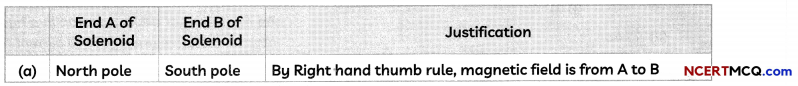(d) End A of Solenoid: South Pole; End B of Solenoid: North Pole; Justification: By Right hand thumb rule, magnetic field is from B to A.

Explanation: The direction of magnetic field around a current carrying conductor is found by using Right Hand Thumb rule, which states that “Imagine that you are holding a current-carrying straight Conductor in your right hand such that the thumb points towards the direction of cur-rent. Then your fingers will wrap around the conductor in the direction of the field lines of the magnetic field”.

We find that the direction of magnetic field is from B to A and as we know that mag-netic field lines originate from North pole and terminate at south pole, we can say that B is North pole and A is South pole.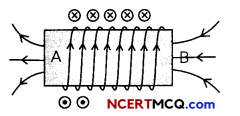Explanation: The magnetic field of a sole-noid increases when we insert an iron core because iron is a ferromagnetic material and ferromagnetic materials help in in-creasing the magnetic property.

(D) Where should a magnetic compass be placed in solenoid to get maximum de-flection in the magnetic compass?
(a) Inside the coil
(b) Near the North Pole
(b) Near the South Pole
(d) In the middle, just above the coil
(E) The magnetic field strength of a sole¬noid can be increased by inserting which of the following materials as the core?
(a) Copper
(b) Silver
(c) Iron
(d) AluminiumQuestion 12.
When Joy went to his friend’s place to complete the group task, he saw that several appliances were connected to the same electric supply point. He asked his friend to remove some of the appliances immediately.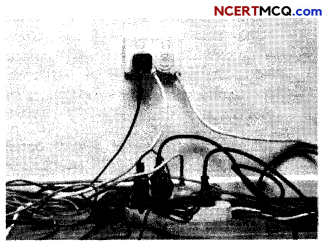What is the danger if too many appliances are connected to the same electric socket?
If too many appliances are connected to the same socket, it may lead to overloading due to which the live and neutral wires may come in contact and cause electric fires.

Question 13.
In Bohr’s model of the hydrogen atom, the electron moves in a circular orbit around the proton. The electron passes by a particular point on the loop in a certain time, so we can calculate a current I = Q/t.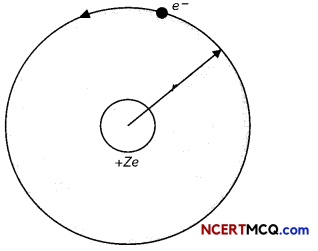through a circular wire. 1/Ve know that a current carrying conductor has a magnetic field associated with it. It is therefore reasonable to conclude that the hydrogen atom produces a magnetic field and interacts with other magnetic fields.

The figure shows the variation of the magnetic field along the axis of a circular loop with distance from the centre.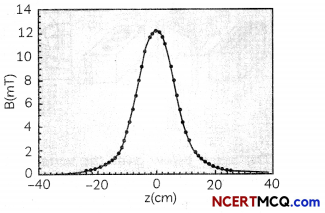(A) Select the correct statements regarding magnetic field produced in a hydrogen atom:
(I) Magnetic field is due to the electrostatic force of attraction between its electron and proton.
(li) Magnetic field is produced in a hydrogen atom as it has no neutron in its nucleus.
(lii) Current is produced in a Hydrogen atom as the electron orbits around the proton.
(IV) Magnetic field is produced in hydrogen atom as this is similar to current flowing through a circular
wire.
(a) Both (I) and (II)
(b) Both (II) and (III)
(c) Both (III) and (IV)
(d) Both (I) and (IV)

(B) Observe the graph between magnetic field along the axis of a circular loop with distance from the centre and select the correct option:
(a) The value of B increases exponentially with distance from the centre on either side of the loop.
(b) The value of B increases linearly with distance from the centre on either side of the loop.
(c) The value of B is minimum at the centre and it increases as we go away from the centre on either side of the loop.
(d) The value of B is maximum at the centre and it decreases as we go away from the centre on either side of the loop.
(d) The value ofB is maximum at the centre and it decreases as we go away from the centre on either side of the Loop.

Explanation: As observed from the graph, the magnetic field along the axis of the circular Loop is maximum at z = 0 cm, which is the centre of the circular loop and decreases as we move away from the centre along the axis.

(C) Select the incorrect statement:
The magnetic field produced by a circular coil of n turns carrying current is:
(a) Inversely proportional to the radius of the circular loop.
(b) Directly proportional to the radius of the circular loop.
(c) Directly proportional to the number of turns in the coil.
(d) Directly proportional to the current in the coil.
(a) Inside the loop: Downward; Outside the loop: Upward; Rule Applied: Right Hand Thumb Rule

Explanation: Applying Right hand thumb rule, we find that the direction of magnetic field inside the loop is in downward direction and in upward direction outside the loop.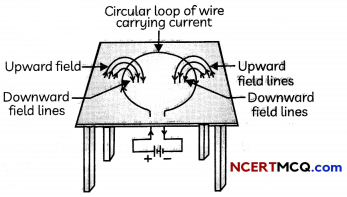(D) Consider a circular loop of wire lying in the plane of the table. Let the current pass through the loop clockwise. Apply the right-hand rule to find out the direction of the magnetic field inside and outside the loop.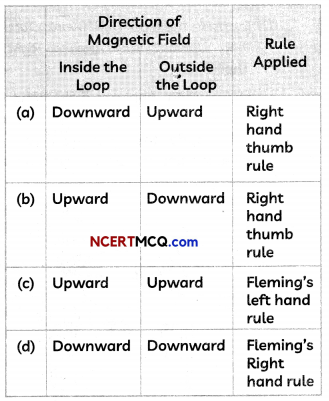(E) The diagram showing the correct direction of the magnetic field lines at point P and Q in the current carrying circular loop is: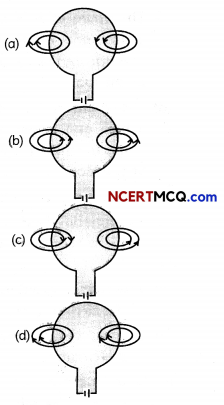Question 14.
Meters, such as those in analog fuel gauges on a car, are common application of magnetic torque on a current-carrying loop. Figure below shows that a meter is very similar in construction to a motor. The torque is proportional to current I. A linear spring exerts a counter-torque that balances the current-produced torque. This makes the needle deflection proportional to I. If an exact proportionality cannot be achieved, the gauge reading can be calibrated.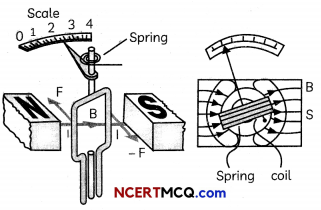The graph below gives the variation of force experienced by a current carrying conductor placed in a magnetic field and the magnitude of current.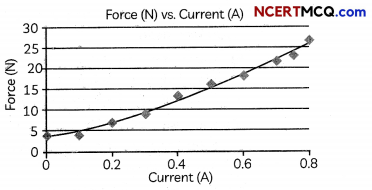(A) The graph above shows that the force experienced by a current carrying conductor in a magnetic field is:
(a) uniform.
(b) Increases linearly with current.
(c) Decreases linearly with current
(d) Reverses on reversing direction of current.
(b) Increases Linearly with current.

Explanation: We observe that the graph between force and current is a straight Line which shows that the force experienced by a current carrying conductor placed in a magnetic field increases linearly with the magnitude of current.

(B) The direction of force experienced by the current carrying loop in the above meter is given by:
(a) Right hand thumb rule
(b) Fleming’s Right hand rule
(d) Fleming’s left hand rule

(C) The magnitude of the force is the highest when the angle between the direction of current and the direction of magnetic field is:
(a) 0°
(b) 45°
(c) 90°
(d) 180°

(D) The relation between direction of current and the direction of force acting on a current carrying conductor when it is ptaced in a magnetic fieLd is:
(a) They are perpendicular to each other
(b) They are parallel to each other
(c) They are opposite to each other
(d) Cannot be predicted
(a) They are perpendicular to each other

Explanation: The direction of force acting on a current carrying conductor, direction of current in the conductor and direction of magnetic field are mutually perpendicular to each other.

(E) Crosses x represent a uniform magnetic field directed into the paper. A conductor XY placed in the field carries current in Y to X direction.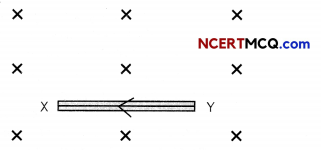The direction of the force experienced by the conductor, direction of force if the direction of field and direction of current both are reversed and name of the rule applied are given below.
Select the row containing the correct directions of force and ruLe appLied.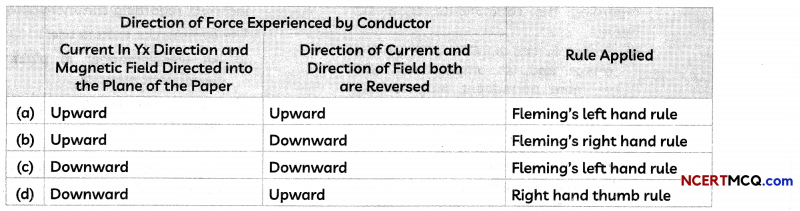(c) direction of force experienced by conductor : Downward, Downward; Rule Applied: fleming’s left hand rule.

Explanation: Applying Fleming’s left hand rule, we find that force acts in downward direction. When the direction of both current and magnetic field are reversed, direction of force will still be in downward direction.Question 15.
DC Motor is a motor which converts DC power into rotary movement and so is the name Direct Current (DC) Motor. It works on the principle of energy conversion i.e. Electrical energy to Mechanical energy. Application of Voltage produces Torque which results in Motion. Internal view of this type of Motor is shown in the Fig. below.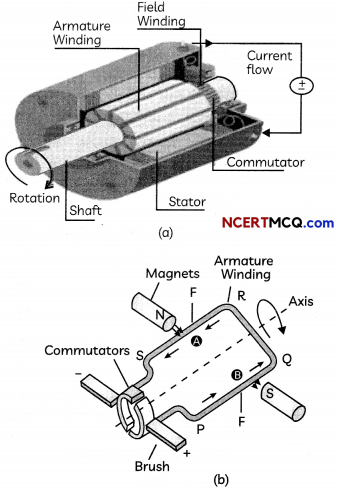It consists of four main components. They are: Stator, Rotor, Winding and Commutator
Stator: The outside part of a motor is called as Stator. It is composed of two or more permanent magnet pole pieces.
Rotor: The inner part of the motor which rotates is called as rotor. It is composed of armature winding which is connected to the external circuit through commutator. Similar to stator, rotor is also made up of ferromagnetic materials.
Winding: Winding consists of series or parallel connection of coils made of copper. Commutator: A commutator acts as a switch that reverses the direction of current between the rotor and external circuit.
(A) Select the incorrect statements regarding the components of a DC motor:
(I) Stator is the outside part of the motor and is composed of two or more permanent magnet pole pieces.
(II) Rotor is the outer part of the motor which rotates and is composed of armature winding.
(III) Winding consists of only parallel connection of coils made of copper.
(IV) Commutator acts as a switch that reverses the direction of current between the rotor and external circuit.
(a) Both (I) and (II)
(b) Both (II) and (III)
(c) Both (III) and (IV)
(d) Both (I) and (IV)

(B) Refer to the figure (b) above and select the row containing the correct directions of the force acting on arms PQ and RS :

 Direction of Force acting on: PQ RS (a) Upwards Downwards (b) Upwards Upwards (c) Downwards Downwards (d) Downwards Upwards

(C) Which of the following rule is used to determine the direction of rotation of D.C motor?
(b) Lenz’s Law
(c) Fleming’s Right-hand Rule
(d) Fleming’s Left-hand Rule

(D) The role of commutator in DC motors is to:
(a) Reverse the direction of current after every half rotation of the coil.
(b) Reverse the direction of current one complete rotation of the coil.
(c) Maintain the same direction of current after one complete rotation of the coil.
(d) To reverse the direction of magnetic field after every half rotation of the coil.

(E) Which of the following statements is incorrect regarding commercial motors?
The commercial motors use:
(a) An electromagnet in place of a permanent magnet.
(b) A permanent magnet in place of an electromagnet.
(c) Large number of turns of the conducting wire in the current carrying coil.
(d) A soft iron core on which the coil is wound.
(E) (b) A permanent magnet in place of an electromagnet.
Explanation: The commercial motors use an electromagnet in place of permanent magnet. Moreover, the soft iron core, on which the coil is wound, plus the coils, is called an armature. This enhances the power of the motor.Question 16.
Generators are useful appliances that supply electrical power during a power outage and prevent discontinuity of daily activities or disruption of business operations. Generators have been a must-have item for vigilant business owners and homeowners for decades. And they’ve only grown more popular in recent years, thanks in part to their wide range of functions. Generators are synonymous with disaster preparedness and emergency use is one of their most common jobs. Generators’ usefulness during a natural disaster such as a hurricane or flood that knocks out power for days on end ranges from convenient to downright life-saving.

There are two main types of backup generators: AC (alternating current) and DC (direct current). AC and DC generators both use electromagnetic induction to generate electricity. However, the process they use is different. An AC generator creates an alternating current that periodically reverses direction. But in a DC generator, a direct current flows in one direction.(A) The rule to determine the direction of induced current in a coil is:
(a) Coulomb’s law
(b) Right hand rule
(c) Fleming’s left hand rule
(d) Fleming’s right hand rule

(B) The following table shows the directions of motion of conductor, magnetic field and current in the Fleming’s right hand rule. Select the row containing the correct combination:(a) Thumb: Motion of conductor; Forefinger: Magnetic field; Middle or Central Finger: Inducent Current

Explanation: Fleming’s right hand rule states that if you stretch the thumb,forefinger and middle finger of the right handmutually perpendicular to each other such thatthe forefinger gives the direction of magneticfield and the thumb points in the direction of themotion of a conductor then, the middle fingerwill give the direction of the induced current.

(C) Select the incorrect statements regarding electric generator:
(I) In an electric generator, electrostatic energy is used to rotate a conductor in a magnetic field to produce electricity.
(II) Electric generator works on the principle of electromagnetic induction.
(III) Current is induced in a coil when a coil is rotated in an electric field.
(IV) Large current is induced if there are larger numbers of turns in the coil.
(a) Both (I) and (II)
(b) Both (I) and (III)
(c) Both (II) and (III)
(d) Both (II) and (IV)

(D) The essential difference between an AC generator and a DC generator is that
(a) AC generator has an electromagnet while a DC generator has permanent magnet.
(b) DC generator will generate a higher voltage.
(c) AC generator will generate a higher voltage,
(d) AC generator has slip rings while the DC generator has a commutator.
(d) AC generator has slip rings while the DC generator has a commutator.

Explanation: To get a direct current a split¬ring type commutator must be used. With this arrangement,one brush is at all times in contact with the arm moving up in the field,while the other is in contact with the arm moving down.Thus a unidirectional current is produced and the generatoris called a DC generator. Whereas, in AC generator, two rings are present.

(E) The meaning of the statement “Frequency of AC in India is 50 Hz” is:
(a) The AC changes direction after every 50 s
(b) The AC changes direction after every 100 s
(c) The AC changes direction after every 1/100 s
(d) The AC changes direction after every 1/50 s
(c) The AC changes direction after every 1/100 s.

Explanation: Frequency is the number of complete oscillations in one second. Frequency of 50 Hz means that there are 50 complete oscillations in 1 second. As AC changes direction twice in one oscillation, it changes direction after every 1/100 s.

Question 1.
Define the term induced electric current.
Induced electric current: It is the current which is created due to the relative motion of coil or magnet. The induced current is found to be the highest when the direction of motion of the coil is at right angles to the magnetic field.

Related Theory
Oersted’s experiments showed that a current produces a magnetic field. Faraday discovered that a moving magnet can be used to generate electric currents.
Induced current is not like the one due to an electric cell.
In an electric cell, the chemical energy is converted into electric energy.
The phenomenon of generation of an electric current in a circuit from magnetic effects is called electro-magnetic induction.

Question 2.
The change in magnetic field lines in a coil is the cause of induced electric current in it. Name the underlying phenomenon.Question 3.
What is the function of a galvanometer in a circuit?
Galvanometer is an electromechanical instrument used to detect or indicate the presence of current by deflection in a circuit.
It consists of a pointer which can move along a scale with zero marked at its centre and is attached to a moving coil.

Related Theory
Depending on the direction of induced current given by Flemings, Right Fiand rule, the pointer of galvanometer can deflect to the right or left of the zero mark of the scale.

If no current is induced, the pointer remains at the centre of the scale, which reads 0.

Question 4.
Why does a compass needle get deflected when brought near a bar magnet?
The needle of a compass is a small magnet. When a compass needle is brought near a bar magnet, its magnetic field lines interact with that of the bar magnet and therefore the compass needle gets deflected.

Question 5.
A straight wire carrying electric current is moving out of a plane of paper and is perpendicular to it. What is the direction of the magnetic field?

Question 6.
What happens when an iron core is inserted into a current carrying solenoid?
When an iron core is inserted into a current carrying solenoid, strength of the magnetic field produced inside the solenoid increases and it forms an electromagnet.Question 7.
Under what condition does a current carrying conductor kept in a magnetic field experience maximum force?